Journal topic
Atmos. Meas. Tech., 12, 3777–3788, 2019
https://doi.org/10.5194/amt-12-3777-2019Atmos. Meas. Tech., 12, 3777–3788, 2019
https://doi.org/10.5194/amt-12-3777-2019Research article 11 Jul 2019

Research article | 11 Jul 2019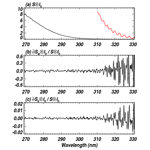# Linearization of the effect of slit function changes for improving Ozone Monitoring Instrument ozone profile retrievals

Linearization of the effect of slit function changes for improving Ozone Monitoring Instrument ozone profile retrievals
Juseon Bak1, Xiong Liu1, Kang Sun2, Kelly Chance1, and Jae-Hwan Kim3 Juseon Bak et al.
• 1Harvard-Smithsonian Center for Astrophysics, Cambridge, MA, USA
• 2Research and Education in Energy, Environment and Water Institute, University at Buffalo, Buffalo, NY, USA
• 3Atmospheric Science Department, Pusan National University, Busan, Korea

Correspondence: Juseon Bak (juseon.bak@cfa.harvard.edu)

Abstract

We introduce a method that accounts for errors caused by the slit function in an optimal-estimation-based spectral fitting process to improve ozone profile retrievals from the Ozone Monitoring Instrument (OMI) ultraviolet measurements (270–330 nm). Previously, a slit function was parameterized as a standard Gaussian by fitting the full width at half maximum (FWHM) of the slit function from climatological OMI solar irradiances. This cannot account for the temporal variation in slit function in irradiance, the intra-orbit changes due to thermally induced change and scene inhomogeneity, and potential differences in the slit functions of irradiance and radiance measurements. As a result, radiance simulation errors may be induced due to convolving reference spectra with incorrect slit functions. To better represent the shape of the slit functions, we implement a more generic super Gaussian slit function with two free parameters (slit width and shape factor); it becomes standard Gaussian when the shape factor is fixed to be 2. The effects of errors in slit function parameters on radiance spectra, referred to as pseudo absorbers (PAs), are linearized by convolving high-resolution cross sections or simulated radiances with the partial derivatives of the slit function with respect to the slit parameters. The PAs are included in the spectral fitting scaled by fitting coefficients that are iteratively adjusted as elements of the state vector along with ozone and other fitting parameters. The fitting coefficients vary with cross-track and along-track pixels and show sensitivity to heterogeneous scenes. The PA spectrum is quite similar in the Hartley band below 310 nm for both standard and super Gaussians, but is more distinctly structured in the Huggins band above 310 nm with the use of super Gaussian slit functions. Finally, we demonstrate that some spikes of fitting residuals are slightly smoothed by accounting for the slit function errors. Comparisons with ozonesondes demonstrate noticeable improvements when using PAs for both standard and super Gaussians, especially for reducing the systematic biases in the tropics and midlatitudes (mean biases of tropospheric column ozone reduced from $-\mathrm{1.4}\sim \mathrm{0.7}$ to 0.0∼0.4 DU) and reducing the standard deviations of tropospheric ozone column differences at high latitudes (by 1 DU for the super Gaussian). Including PAs also makes the retrievals consistent between standard and super Gaussians. This study corroborates the slit function differences between radiance and irradiance, demonstrating that it is important to account for such differences in the ozone profile retrievals.

1 Introduction

The fitting of measured spectra to simulated spectra is the most basic concept for analysis of the Earth's atmospheric constituents from satellite measurements. Therefore, accurate calibration and simulation of measurements are essential for the successful retrieval of atmospheric constituents. The knowledge of the instrumental spectral response function (ISRF) or slit function could affect the accuracies of both calibration and simulation, as it is required for the convolution of a high-resolution reference spectrum to the instrument's spectral resolution in the wavelength calibration and for the convolution of high-resolution absorption cross section spectra or simulated radiance spectra in the calculation of radiance at instrumental resolution. Compared to other trace gases, the retrieval of ozone profiles can be more susceptible to the accuracy of ISRFs due to the large spectral range, where the radiance spans a few orders of magnitude, and to the fact that the spectral fingerprint for the tropospheric ozone is primarily provided by the 310–330 nm absorption features residing in the temperature-dependent Huggins bands. Therefore, the efforts to characterize and verify the ISRFs have preceded the analyses of ozone profiles from satellite and aircraft measurements (X. Liu et al., 2005, 2010; Cai et al., 2012; C. Liu et al., 2015; Sun et al., 2017; Bak et al., 2017).

As such, the objective of this paper is to expand the slit function linearization proposed by Beirle et al. (2017) into the optimal-estimation-based spectral fitting of the SAO ozone profile algorithm. The slit function linearization is used to account for the radiative transfer calculation errors caused by the slit functions differences between radiance and irradiance on a pixel-by-pixel basis, and ultimately to improve OMI ozone profile retrievals. This paper is organized as follows: after a mathematical description of the linearization of slit function changes using the generic super Gaussian function, we introduce their practical application in an optimal estimation based spectral fit procedure (Sect. 2). This linearization scheme is implemented differently, depending on the simulation scheme of measured spectra using high resolution or effective cross section data, respectively. Section 3 characterizes the derived pseudo absorber spectra, along with evaluations of ozone profile retrievals using independent ozonesonde observations as a reference dataset. Finally, the summary of this study is given in Sect. 4.

2 Method

## 2.1 Super Gaussian linearization

The slit function parameterization and linearization are briefly summarized as in Beirle et al. (2017), focusing on what we need to derive the pseudo absorbers in the terms of the optimal estimation based fitting process. The slit function can be parameterized with the slit width w and shape factor k assuming the super Gaussian, S, to be

$\begin{array}{}\text{(1)}& S\left(\mathrm{\Delta }\mathit{\lambda }\right)=A\phantom{\rule{0.125em}{0ex}}\left(w,\phantom{\rule{0.125em}{0ex}}k\right)\phantom{\rule{0.125em}{0ex}}×\phantom{\rule{0.125em}{0ex}}exp\left[-\phantom{\rule{0.125em}{0ex}}{\left|\frac{\mathrm{\Delta }\mathit{\lambda }}{w}\right|}^{k}\right],\end{array}$

where A(w, k) is $\frac{k}{\mathrm{2}{\mathit{\sigma }}_{g}\mathrm{\Gamma }\left(\frac{\mathrm{1}}{w}\right)}$ with Γ representing the gamma function. This equation allows many forms of distributions by varying k: the top-peaked function (k< 2), the standard Gaussian function (k=2), and the flat-topped function (k> 2). w is converted to the full width at half maximum (FWHM) via the relationship of $\mathrm{FWHM}=\mathrm{2}\sqrt[k]{\mathrm{ln}\mathrm{2}}\phantom{\rule{0.125em}{0ex}}w$ . We investigate the impact of including one more slit parameter k on the OMI ISRF fitting results over the standard Gaussian using OMI daily solar measurements. As an example, time series (2005–2015) of the fitted slit width and shape factor in 310–330 nm are displayed in Fig. 1a. The FWHM and shape factor of the super Gaussian function is on average 0.44 nm and 2.9, respectively, while the FWHM of the standard Gaussian is 0.395 nm. The sharp change and random-noise of these derived slit function parameters might be influenced by the decreasing signal-to-noise ratio (SNR) of solar spectra later in the OMI mission and radiometric errors in solar irradiance due to the row anomaly (Sun et al., 2017). Figure 1b illustrates the high wavelength stability (0.003 nm) in the OMI mission, verifying that better calibration stability is performed with super Gaussian slit functions as abnormal deviations of wavelength shifts are derived with standard Gaussian slit functions.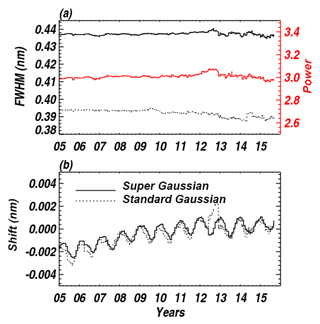Figure 1Time series of (a) slit parameters and (b) wavelength shifts for OMI daily irradiance measurements (310–330 nm) at nadir cross-track position when super Gaussians (solid line) and standard Gaussians (dotted line) are parameterized as slit function shapes.

The effect of changing the slit parameters p on the slit function can be linearized by the first-order Taylor expansion approximation around So=S(po):

$\begin{array}{}\text{(2)}& S=S-{S}_{\mathrm{o}}\approx p\frac{\partial S}{\partial p},\end{array}$

and thus the effect of changes in S on the convolved high-resolution spectrum can be parameterized as

$\begin{array}{}\text{(3)}& I=I-{I}_{\mathrm{o}}=S\phantom{\rule{0.125em}{0ex}}\otimes \phantom{\rule{0.125em}{0ex}}{I}_{\mathrm{h}}-{S}_{\mathrm{o}}\phantom{\rule{0.125em}{0ex}}\otimes \phantom{\rule{0.125em}{0ex}}{I}_{\mathrm{h}}=S\phantom{\rule{0.125em}{0ex}}\otimes \phantom{\rule{0.125em}{0ex}}{I}_{\mathrm{h}},\end{array}$

where the convolved spectrum is $I=S\phantom{\rule{0.125em}{0ex}}\otimes \phantom{\rule{0.125em}{0ex}}{I}_{\mathrm{h}}$. Consequently, the partial derivatives of I with respect to slit parameters p are defined as

$\begin{array}{}\text{(4)}& \frac{\partial I}{\partial p}=\frac{\partial S}{\partial p}\phantom{\rule{0.125em}{0ex}}\otimes \phantom{\rule{0.125em}{0ex}}{I}_{\mathrm{h}}.\end{array}$

In Beirle et al. (2017) $\frac{\partial I}{\partial p}$ is referred to as Jp, resolution correction spectra (RCS). In Fig. 2, we present an example of Jp over the typical ozone profile fitting range (270–330 nm) through the convolution of high-resolution ozone cross sections (δh) with the derivatives of the super Gaussian $\left(\frac{\partial S}{\partial p}\right)$. The baseline So is defined with w=0.26 nm and k=2.6, which are averaged parameters from climatological OMI solar irradiance spectra in the UV2 band (310–330 nm). Note that this w value corresponds to a FWHM of 0.45 nm. The change in the assumed OMI slit function causes a highly structured spectral response over the whole fitting window. However, the relative magnitude of the responses with respect to both slit parameters is more distinct in the Huggins band (> 310 nm) where narrow absorption features are observed as shown in Fig. 2a. An anticorrelation (0.92) is found between $\frac{\partial \mathrm{ln}\mathit{\delta }}{\partial w}$ and $\frac{\partial \mathrm{ln}\mathit{\delta }}{\partial k}$ while the response of the unit change of the slit width to the convolved spectrum is dominant against that of the shape factor.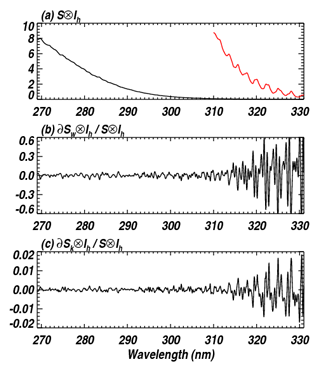Figure 2(a) Ozone absorption cross sections (cm2 mol−1) (δh) at different scales (red and black) at a representative temperature (238.12 K) calculated via convolution of a high-resolution (0.01 nm) reference spectrum with the super Gaussian slit function, S (k=26, w=026 nm). (b–c) Its derivatives with respect to slit parameters $\left(\partial {S}_{p}=\frac{\partial }{\partial p}\right)$, w and k, normalized to the convolved cross sections.

## 2.2 Implementation of the slit function linearization in the SAO ozone profile algorithm

In Beirle et al. (2017) a slit function linearization was implemented only to fit solar irradiances from GOME-2. We implement the slit function linearization to fit radiances in the SAO ozone profile algorithm (X. Liu et al., 2010), which is routinely being performed to produce the OMI PROFOZ product (https://avdc.gsfc.nasa.gov/, last access: 7 July 2019). Two spectral windows (270–309 nm in the UV1 band and 312–330 nm in the UV2 band) are employed to retrieve ozone profiles from OMI BUV measurements. To match the different spatial resolutions between UV1 and UV2 bands, every two cross-track pixels are averaged for the UV2 band, resulting in 30 positions with the spatial resolution of 48 km (across-track) × 13 km (along-track) at nadir position. Partial ozone columns at 24 layers between the surface and 60 km are iteratively estimated toward minimizing the fitting residuals between measured and simulated radiances and simultaneously between a priori and estimated ozone values using the optimal estimation inversion method. A priori ozone information is taken from a tropopause-based (TB) ozone profile climatology (Bak et al., 2013). The vector linearized discrete ordinate radiative transfer model (VLIDORT; Spurr, 2008) is used to simulate the radiances and their derivatives with respect to geophysical parameters. The radiance calculation is made for the Rayleigh atmosphere, where the incoming sunlight is simply absorbed by ozone and other trace gases, scattered by air molecules, and reflected by surfaces/clouds assumed as a Lambertian surface. In addition to these, other physical phenomena are treated as PAs to the spectral response such as Ring effect, additive offset, and spectral shifts due to misalignments of radiance relative to irradiance and ozone cross sections. In the SAO algorithm, these PAs are derived using finite differences of the radiances with and without perturbation to a phenomenon, except for the Ring spectrum that is calculated using a first-order single-scattering rotational Raman scattering model (Sioris and Evans, 2000). In this paper, we introduce new PAs to account for the radiance simulation errors caused by the slit function errors. The OMI ISRFs have been parameterized as a standard Gaussian from climatological OMI solar irradiances for each UV1 and UV2 band and thereby these PAs could take into account the spectral fitting responses caused by temporal variations in the slit function. This ozone fitting procedure uses ISRFs to convolve high-resolution absorption spectra, taken from Brion et al. (1993) for ozone absorption cross sections and Wilmouth et al. (1999) for BrO absorption cross sections. In DOAS analysis, the pseudo absorber is defined as $\frac{\partial S}{\partial p}\phantom{\rule{0.125em}{0ex}}\otimes \phantom{\rule{0.125em}{0ex}}{\mathit{\sigma }}_{\mathrm{h}}$ (σh is a high-resolution absorption cross section), which could be calculated at a computationally low cost. In our optimal-estimation-based ozone profile retrievals, it is conceptually defined as $\frac{\partial S}{\partial p}\phantom{\rule{0.125em}{0ex}}\otimes \phantom{\rule{0.125em}{0ex}}{I}_{\mathrm{h}}$ (Ih is a high-resolution simulated radiance), which is computationally very expensive because of online radiative calculation for a ∼60 nm wide fit window on the spatial pixel-to-pixel basis. We now introduce how to implement the slit function linearization to derive the derivatives of the OMI radiances with respect to slit function changes in two different radiative transfer approaches used in the SAO ozone profile algorithm, i.e., the effective cross section approach in X. Liu et al. (2010) and the updated high-resolution convolution approach described in Kim et al. (2013).

In X. Liu et al. (2010), VLIDORT simulates the radiances at OMI spectral grids (λomi) using effective cross sections that are produced by convolving high-resolution cross sections with the OMI ISRFs. Therefore, we apply a similar convolution process of matching the high-resolution cross section spectra with OMI spectra to derive the partial derivative of σx with respect to slit parameter p as follows:

$\begin{array}{}\text{(5)}& \frac{\partial {\mathit{\sigma }}_{x}}{\partial p}=\frac{\partial S}{\partial p}\phantom{\rule{0.125em}{0ex}}\otimes {\mathit{\sigma }}_{x,\mathrm{h}},\end{array}$

where σx,h is a high-resolution absorption spectrum for ozone or BrO. Due to the dominant absorption of O3 over BrO, the derivative of the BrO cross section with respect to p is neglected here. This partial derivative of ozone is then converted to the partial derivative of radiance through the chain rule with the analytical ozone weighting function ($\frac{\mathrm{dln}I}{{\mathrm{dO}}_{\mathrm{3}}}$), calculated from VLIDORT, as follows:

$\begin{array}{}\text{(6)}& \frac{\partial \mathrm{ln}I}{\partial p}=\frac{\partial \mathrm{ln}I}{\partial {\mathrm{O}}_{\mathrm{3}}}\phantom{\rule{0.125em}{0ex}}\phantom{\rule{0.125em}{0ex}}\frac{\partial \mathit{\sigma }}{\partial p}\phantom{\rule{0.125em}{0ex}}\frac{{\mathrm{O}}_{\mathrm{3}}}{\mathit{\sigma }}.\end{array}$

This simulation process is hereafter referred to as effective resolution cross section (ER) simulation.

As described in Kim et al. (2013), the radiative transfer calculation in the SAO ozone profile algorithm has been performed using high-resolution extinction spectra at the optimized sampling intervals for resolving the ozone absorption features, which are ∼1.0 nm below 300 nm and ∼0.4 nm above 300 nm. These sampling intervals are coarser than actual OMI sampling grids with approximately half the number of wavelengths. The coarser sampled simulated radiances are then interpolated to a fine grid of 0.05 nm assisted by the weighting functions with respect to absorption and Rayleigh optical depth:

$\begin{array}{}\text{(7)}& \begin{array}{rl}& I\left({\mathit{\lambda }}_{\mathrm{h}}\right)=I\left({\mathit{\lambda }}_{c}\right)+\frac{\partial I\phantom{\rule{0.125em}{0ex}}\left({\mathit{\lambda }}_{c}\right)}{\partial {\mathrm{\Delta }}_{\mathrm{l}}^{\mathrm{gas}}}\left({\mathrm{\Delta }}_{\mathrm{l}}^{\mathrm{gas}}\left({\mathit{\lambda }}_{\mathrm{h}}\right)-{\mathrm{\Delta }}_{\mathrm{l}}^{\mathrm{gas}}\left({\mathit{\lambda }}_{\mathrm{c}}\right)\right)\\ & \phantom{\rule{0.25em}{0ex}}\phantom{\rule{0.25em}{0ex}}+\frac{\partial I\phantom{\rule{0.125em}{0ex}}\left({\mathit{\lambda }}_{\mathrm{c}}\right)}{\partial {\mathrm{\Delta }}_{\mathrm{l}}^{\mathrm{ray}}}\left({\mathrm{\Delta }}_{\mathrm{l}}^{\mathrm{ray}}\left({\mathit{\lambda }}_{\mathrm{h}}\right)-{\mathrm{\Delta }}_{\mathrm{l}}^{\mathrm{ray}}\left({\mathit{\lambda }}_{\mathrm{c}}\right)\right)\end{array},\end{array}$

where ${\mathrm{\Delta }}_{\mathrm{l}}^{\mathrm{gas}}$ and ${\mathrm{\Delta }}_{\mathrm{l}}^{\mathrm{ray}}$ are the optical thickness (the product of cross section and layer column density) at each layer for trace gas absorption and Rayleigh scattering, respectively. The convolution is then applied to these simulated high-resolution radiances I(λh) with assumed slit functions and derivatives and thereby I(λomi) and $\frac{\partial \mathrm{ln}I}{\partial p}$ are calculated. This simulation process is hereafter referred to as high-resolution cross section (HR) simulation. The ER simulation is more commonly implemented in trace gas retrievals in the UV and visible ranges, but the HR simulation allows for more accurate fitting residuals, to better than 0.1 % (Kim et al., 2013) as well as shorter computation time. $\frac{\partial \mathrm{ln}I}{\partial p}$ is scaled by the fitting coefficients, Δp to account for the actual size of the spectral structures caused by the slit function differences between radiance and irradiance spectra. The pseudo absorber (PA) for the super Gaussian slit function linearization is expressed as

$\begin{array}{}\text{(8)}& \mathrm{PA}=\phantom{\rule{0.125em}{0ex}}\partial \mathrm{ln}I=\frac{\partial \mathrm{ln}I}{\partial k}\phantom{\rule{0.125em}{0ex}}\mathrm{\Delta }k\phantom{\rule{0.125em}{0ex}}+\phantom{\rule{0.125em}{0ex}}\frac{\partial \mathrm{ln}I}{\partial w}\mathrm{\Delta }w.\end{array}$

In the form of the logarithm of normalized radiance, PA is physically related to the optical depth change Δτ. Figure 3 compares the partial derivatives of radiances to slit parameters $\frac{\mathrm{dln}I}{\mathrm{d}p}$ in HR and ER simulations. Little difference is found even though convolution error for ozone cross sections is only accounted for in the ER simulation due to the overwhelming impact of ozone cross section convolution errors over other cross section data. The amplitude of $\frac{\mathrm{dln}I}{\mathrm{d}p}$ varies with different satellite pixels (e.g., ozone profile shape, geometry, and cloud/surface property), but the spectral peak positions do not change because they arise from the errors due to the convolution process of high-resolution absorption cross sections dominated by ozone. It should be noted that these spectral structures are weakly correlated with the partial derivatives of radiances with respect to other state vectors (ozone, BrO, cloud fraction, surface albedo, radiance–irradiance shift, radiance–ozone cross section shift, Ring, mean fitting residual scaling factor) within ±0.3 and ±0.1 in the UV1 and UV2, respectively.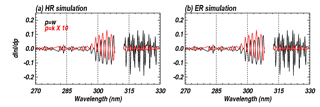Figure 3Derivatives of an OMI radiance spectrum simulated using high-resolution (HR) and effective-resolution (ER) cross section spectra with respect to slit parameters assuming a super Gaussian function. dlnI∕dk is multiplied by a factor of 10 to visually match dlnI∕dw on the same y axis.

Furthermore, this linearization process can be formulated with n-order polynomial fitting parameters pi) to account for the wavelength-dependent change in the slit parameters around a central wavelength $\stackrel{\mathrm{‾}}{\mathit{\lambda }}$ , which is expressed as

$\begin{array}{}\text{(9)}& \begin{array}{rl}& \mathrm{PA}=\frac{\partial \mathrm{ln}I}{\partial k}{\sum }_{i=\mathrm{1}}^{n}\mathrm{\Delta }{k}_{i}\cdot {\left(\mathit{\lambda }-\stackrel{\mathrm{‾}}{\mathit{\lambda }}\right)}^{n-\mathrm{1}}\\ & \phantom{\rule{0.25em}{0ex}}\phantom{\rule{0.25em}{0ex}}+\phantom{\rule{0.125em}{0ex}}\frac{\partial \mathrm{ln}I}{\partial w}{\sum }_{i=\mathrm{1}}^{n}\mathrm{\Delta }{w}_{\mathrm{i}}\cdot {\left(\mathit{\lambda }-\stackrel{\mathrm{‾}}{\mathit{\lambda }}\right)}^{n-\mathrm{1}}.\end{array}\end{array}$
3 Results and discussion

We characterize the effect of including the PA $\left(\frac{\partial \mathrm{ln}I}{\partial p}\cdot p\right)$ on ozone profile retrievals using both super Gaussian and standard Gaussian slit functions. Hereafter, the correction spectrum $\left(\frac{\partial \mathrm{ln}I}{\partial p}\right)$ is derived using the HR simulation. The PA coefficient (Δpi) (one for each channel and for each order) is included as part of the state vector to be iteratively and simultaneously retrieved with ozone. The a priori value is set to be zero for all fitting coefficients, while the a priori error is set to be 0.1, empirically. We should note that the empirical “soft calibration” is applied to OMI radiances before the spectral fitting, in order to eliminate the wavelength- and cross-track-dependent systematic biases, due to the interference of the PA coefficients with systematic measurement errors during the fitting process.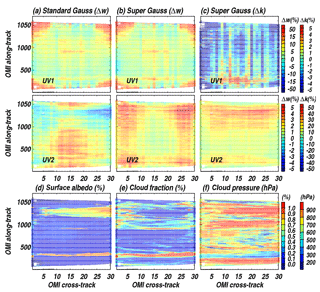Figure 4Pseudo absorption coefficients (Δw, Δk) for fitting OMI radiances to account for slit function changes assuming a (a) standard Gaussian and (b–c) super Gaussian, for the first orbit of measurements on 1 July 2006, with (d–f) the corresponding geophysical parameters. Δw and Δk are displayed after being normalized with wo and ko, the slit parameters derived from OMI solar irradiance measurements.

## 3.1 Characterization of the pseudo absorbers in ozone fitting procedure

Figure 4 displays how the zero-order PA coefficients (Δp) vary within one orbit when slit functions are assumed to be standard and super Gaussians along with variation in cloud fraction, surface albedo, and cloud pressure from the retrievals. These retrieved coefficients physically represent the deviation of ISRFs in radiances from those in solar measurements. We normalize them with the slit parameters derived from OMI solar irradiances for a better interpretation. Cross-track-dependent features are shown in slit width. The relative change in the slit width is more distinct in the UV1 band than in the UV2 band, whereas the change in the shape factor is more distinct in the UV2 band. The UV2 slit widths increase typically within 5 % over the given spatial domain. However, the UV1 slit widths increase from 10 % at most pixels up to 50 % at off-nadir positions in the high latitudes, which might be caused by stray light differences between radiance and irradiance and intra-orbit instrumental changes. An abnormal change in the UV1 slit parameters due to the scene heterogeneity is detected at the along-track scan positions of ∼300 and 900 where upper-level clouds are present. The UV2 shape factor changes show a coherent sensitivity to bright surfaces under clear-sky conditions over the northern high latitudes. Fitting coefficients for the standard Gaussian show a quite similar spatial variation for the UV1 slit width (correlation =∼0.98), but an anticorrelation of $\sim -\mathrm{0.62}$ for the UV2 slit width compared to that for a super Gaussian due to the interference between shape factor and slit width.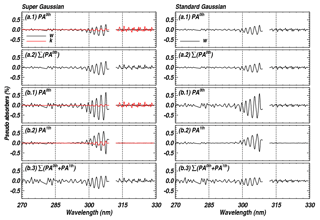Figure 5(a1) Pseudo absorber spectra multiplied by corresponding zero-order coefficients $\frac{\partial \mathrm{ln}I}{\partial p}\phantom{\rule{0.125em}{0ex}}×\phantom{\rule{0.125em}{0ex}}\mathrm{\Delta }{p}_{\mathrm{o}}$ and (a2) the sum of them for (left) super Gaussian and (right) standard Gaussian function parameterizations. Panel (b) is the same as (a), but for first-order polynomial coefficients, $\frac{\partial \mathrm{ln}I}{\partial p}\phantom{\rule{0.125em}{0ex}}×\phantom{\rule{0.125em}{0ex}}\mathrm{\Delta }{p}_{i}\phantom{\rule{0.125em}{0ex}}{\left(\mathit{\lambda }-\stackrel{\mathrm{‾}}{\mathit{\lambda }}\right)}^{i}\left(i=\mathrm{0},\mathrm{1}\right)$. This example represents an average at nadir in the latitude zone 30–60 N from measurements used in Fig. 4.

Examples of the PAs (Eq. 9) are illustrated in Fig. 5 when (a) zero- and (b) first-order polynomial coefficients are fitted. In the UV1 range, the sum of PAs multiplied by corresponding coefficients, regardless of which Gaussian is assumed as the slit function, is very similar because the spectral structure caused by the slit width change is dominant. It implies that OMI ISRFs in the UV1 band are similar to the standard Gaussian, for both radiance and irradiance measurements, consistent with the pre-launch characterization (Dirksen et al., 2006). However, in the UV2 range, the spectral structures are generated by the shape factor change rather than the slit width change and therefore PAs show noticeable discrepancies for different Gaussian assumptions. Our results indicate that the PA for the shape factor change is required to adjust the spectral structures due to the differences in the slit functions between radiance and irradiance over the UV2 band. In the case of the wavelength-dependent PA coefficient fit, the impact of first-order PAs on OMI radiances is relatively visible in the wavelength range of 300–310 nm. This result is physically consistent with the wavelength-dependent property shown in the slit parameters derived from OMI irradiances as shown in Fig. 6 where slit parameters are characterized in 10-pixel increments assuming the super Gaussian slit function. In UV1, the slit widths plotted as FWHM slightly decrease by ∼0.1 nm at shorter wavelengths than 288 nm, but vary more sharply by up to ∼0.2 nm at longer wavelengths. Compared to slit widths, the wavelength dependences of the shape factors are less noticeable, except at boundaries of the window. In the UV2 window, both slit width and shape factor are highly invariant.

## 3.2 Impact of including pseudo absorbers on ozone profile retrievals

Figures 7 to 9 evaluate the impact of including zero-order PAs on ozone profile retrievals. Figure 7 illustrates how different assumptions in the slit functions affect the ozone profile retrievals with respect to the retrieval sensitivity and the fitting accuracy from the case shown in Fig. 4. In this figure, the degrees of freedom for signal (DFS) represent the independent pieces of ozone information available from measurements, which typically decreases as ozone retrievals are further constrained by other fitting variables. The reduced DFS values (< 5 %) imply that the ozone retrievals are correlated slightly with PAs. The fitting accuracy is assessed as the root mean square (rms) of the relative differences ( %) between measured and calculated radiances over the UV1 and UV2 ranges. Including the PAs makes little difference in the UV1 fitting residuals for most of individual pixels (1 %–5 %), but significantly reduces residuals in the UV2 range. The adjusted amounts of the residuals with PAs are generally larger when assuming super Gaussian slit functions. This comes from different assumptions for slit functions in deriving soft calibration spectra, where slit functions were parameterized as standard Gaussians. Therefore, applying soft calibration to OMI spectra entails somewhat artificial spectral structures if ISRFs are assumed as super Gaussian in ozone retrievals, and hence the impact of PAs on the spectral fitting becomes more considerable. Figure 8 compares how the spectral residuals are adjusted with PAs when soft calibration is turned on and off. Using super Gaussians causes larger amplitudes of the spectral fitting residuals than using standard Gaussians, if soft calibration is turned on and PAs are excluded. Conversely, some residuals are reduced and more broadly structured if soft calibration is turned off. Including PAs eliminates or reduces some spikes of fitting residuals as well as improves the consistency of the fitting accuracy between using standard and super Gaussians at wavelengths above 300 nm.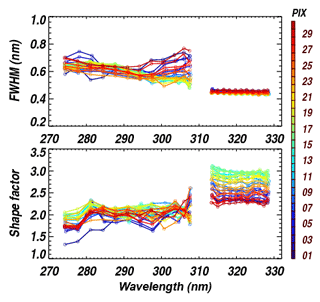Figure 6OMI ISRF FWHM (nm) and shape factor (k) as functions of the center wavelength, as derived from OMI solar irradiances assuming super Gaussian functions over a range of 31 spectral pixels in 10-pixel increments. Different colors represent different cross-track positions from 1 (blue) to 30 (red).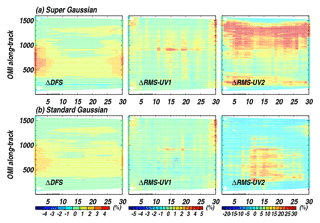Figure 7Same as Fig. 4, but for comparisons of the degrees of freedom for signal (DFS) and the root mean square (rms) of spectral fitting residuals in UV1 and UV2 with and without a zero-order pseudo absorber. Positive values indicate that both fitting residuals and DFSs are reduced due to the pseudo absorber.

The benefit of this implementation for ozone retrievals is further assessed through comparison with electrochemical concentration cell (ECC) ozonesondes collected from the WOUDC (https://woudc.org/, last access: 7 July 2019) and SHADOZ (https://tropo.gsfc.nasa.gov/shadoz/, last access: 7 July 2019) networks. This evaluation is limited to the period of 2005 through 2008 to avoid interferences with row-anomaly effects appearing in 2007 and becoming serious in early 2009 (Schenkeveld et al., 2017). We select 13 SHADOZ sites in the tropics and 38 WOUDC sites in the northern midlatitudes to high latitudes. The collocation criteria are within ±1 in latitude and longitude and within 12 h in time. For comparison, high-vertical-resolution (∼100 nm) profiles of ozonesondes are interpolated onto OMI retrieval grids (∼2.5 km thick). We limit OMI–ozonesonde comparisons to OMI solar zenith angle < 85, effective cloud fraction < 0.4, surface albedo < 20 % (100 %) in the tropics and midlatitudes (high latitude), top altitude of ozonesondes > 30 km, ozonesonde correction factors ranging from 0.85 to 1.15 if they exist, and data gaps for each ozonesonde no greater than 3 km. Comparisons between OMI and ozonesondes are performed for the tropospheric ozone columns (TCOs) over three different latitude bands and for ozone profiles including all the sites, with and without PAs (zero order) for standard and super Gaussian slit function changes.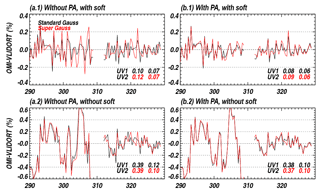Figure 8Average differences ( %) between measured (OMI) and simulated (VLIDORT) radiances at the nadir cross-track pixel in the tropics (30–30 S) from measurements used in Fig. 4, without (a) and with (b) zero-order pseudo absorbers (PA) when the standard Gaussian (black line) and the super Gaussian (red line) are assumed as ISRFs, respectively. Upper/lower panels represent the fitting results with soft calibration turned on/off. The residuals in the UV1 (< 310 nm) are scaled by a factor of 2 to fit on the given y axis. In the legend, the rms of residuals ( %) is given for UV1 and UV2 wavelength ranges.

In Table 1, the comparison statistics of tropospheric ozone columns between OMI and ozonesonde are summarized as a function of latitude bands. Without using PAs, the comparison results show a noticeable discrepancy in mean biases (1.3–2.1 DU or 3.9 %–6.4 %) due to different assumptions on the slit function shape, with positive biases of 0.3–0.7 DU for super Gaussians and negative biases of 1.0–1.4 DU for standard Gaussians. Overall, OMI retrievals are in a better agreement with ozonesonde measurements using super Gaussians. The correlations and standard deviations are very similar in the tropics and midlatitudes, but the retrievals with standard Gaussians show better correlation and smaller standard deviations at high latitudes. As in Sun et al. (2017), the retrievals show significant differences between using standard and super Gaussians, although there are some inconsistencies in comparing OMI and ozonesondes; the main inconsistent factors are as follows. In this study, soft calibration is turned on and a priori information is taken from the TB climatology to perform OMI ozone profile retrievals, whereas soft calibration is turned off and a priori information is taken from the Labow–Logan McPeters (LLM) climatology (McPeters et al., 2007) in Sun et al. (2017). OMI and ozonesonde data filtering criteria are quite similar to each other, except that the criteria of the solar zenith angle and cloud fraction are relaxed from 75 and 0.3 to 85 and 0.4, respectively, and the adjustment of ozonesondes with correction factors given for the WOUDC dataset is turned on in this study. Comparison is performed by latitudes here whereas global comparison is analyzed in Sun et al. (2017). After accounting for the slit differences between radiances and irradiances using PAs, the retrievals are improved for both standard and super Gaussians and these two retrievals become consistent except for the use of super Gaussians in the tropics. The mean biases in the tropics and midlatitudes are almost eliminated, but the standard deviations and correlation do not change much. In the high latitudes, the standard deviations and correlation are significantly improved due to applying PAs with super Gaussian ISRFs. The lack of improvement with PAs in the tropics with super Gaussians illustrates that ISRFs of radiances are quite similar to those of irradiances in the tropics, while super Gaussians better parameterize OMI ISRFs than standard Gaussians. This is consistent with the comparison of the fitting accuracy of the UV2 band as shown in Fig. 7, where the fitting residuals are slightly reduced in the tropics when super Gaussians are linearized, but the standard Gaussian linearization significantly improves the fitting accuracy. The mean biases of the profile comparison as shown in Fig. 9 clearly show that including PAs to account for ISRF differences reduces mean biases by up to ∼5 % below 10 km and their general altitude dependence and improves the consistency between using standard and super Gaussians; in addition, the standard deviations are slightly improved in the 10–20 km altitude range for both Gaussians. The improvement at all latitudes corroborates the change in ISRFs between radiance and irradiance along the orbit as conjectured by Sun et al. (2017). The consistency between using standard and super Gaussians after using PAs is mainly because there is strong anticorrelation between the slit width and shape partial derivatives as shown in Fig. 2, so the adjustment of slit width only in the use of standard Gaussians can achieve almost the same effect as the adjustment of both parameters in the use of super Gaussians. Accounting for the wavelength-dependent change in the ISRFs with first-order PAs makes insignificant differences to both fit residuals and ozone retrievals (not shown here). This could be mainly explained by negligible wavelength dependence of OMI ISRFs especially in UV2 as shown in Fig. 5, where the PA spectrum ($\frac{\partial \mathrm{ln}I}{\partial p}\cdot \mathrm{\Delta }p$) shows almost no variance except at the upper boundary of UV1, as well as in Fig. 6 where the UV2 slit parameters derived from irradiances in the sub-fit windows vary within 0.05 nm for FWHM and 0.2 for shape factor.

Table 1Comparison statistics (mean bias and 1σ standard deviation of absolute (DU) and relative (%) differences, the Pearson correlation coefficient, number of collocations) of OMI and ozonesonde tropospheric column ozone from 2005 to 2008 over (a) tropical, (b) midlatitude, and (c) high-latitude stations.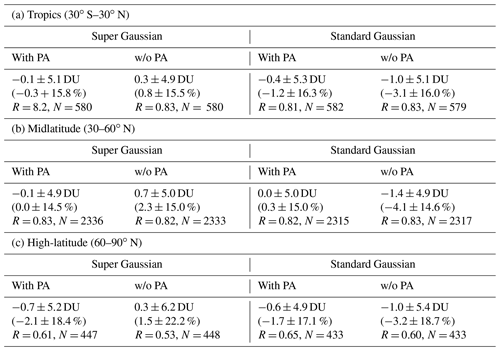4 Summary

Knowledge of the instrument spectral response functions (ISRFs) or slit functions is important for ozone profile retrievals from the Hartley and Huggins bands. ISRFs can be measured in the laboratory prior to launch, but they have been typically derived from solar irradiance measurements assuming Gaussian-like functions in order to account for the effect of the ISRF changes after launch. However, the parameterization of the ISRFs from solar irradiances could be inadequate for achieving a high accuracy of the fitting residuals as ISRFs in radiances could significantly deviate from those in solar radiances (Beirle et al., 2017) and might affect ozone profile retrievals as suggested in Sun et al. (2017). Therefore, this study implements a linearization scheme to account for the spectral errors caused by the ISRF changes as pseudo absorbers (PAs) in an optimal-estimation-based fitting procedure for retrieving ozone profiles from OMI BUV measurements using the SAO ozone profile algorithm. The ISRFs are assumed to be the generic super Gaussian that can be used as standard Gaussians when fixing the shape factor to 2. This linearization was originally introduced in Beirle et al. (2017) for DOAS analysis, but this study extends this application and provides more details on how to implement in practice using two different approaches to derive radiance errors from slit function partial derivatives with respect to slit parameters. These two approaches correspond to the two methods of simulating radiances at instrument spectral resolution, one using effective cross sections, which were previously used in the SAO ozone profile algorithm and are still used in most of the trace gas retrievals from the UV and visible, and the other calculating radiances at high resolution before convolution, which is the preferred method in the SAO ozone profile algorithm. Consistent PAs are derived with these two approaches, as expected.

The fitting coefficients (Δp) to the PAs, representing the difference of slit parameters between radiance and irradiance, are iteratively fitted as part of the state vector along with ozone and other parameters. The UV1 slit parameters show distinct cross-track-dependent differences, especially in high latitudes. In addition, an abnormal Δp caused by scene heterogeneity is observed around bright surfaces and cloudy scenes. The PA spectrum $\left(\frac{\partial I}{\partial p}\cdot \mathrm{\Delta }p\right)$ illustrates that the slit width change causes most of the spectral structures in the UV1 band because the OMI ISRFs are close to Gaussian. Otherwise, the ISRF change results in different spectral responses in the UV2 band with different Gaussian functions because the adjustment of the shape factor becomes more important in accounting for the convolution error when using super Gaussians.

Insignificant wavelength dependence on OMI slit functions is demonstrated from slit function parameters derived from irradiances in the sub-fit window, which leads to little difference in ozone profile retrievals when zero- and first-order wavelength-dependent PA coefficients are implemented to fit the spectral structures caused by slit function errors. Therefore we evaluate the benefit of including the zero-order PA fit for both the accuracy of the fitting residuals and the quality of retrieved ozone profiles through validation against ozonesonde observations. Some spikes in the fitting residuals are reduced or eliminated. Commonly, including PAs makes little change in both fit residuals and ozone retrievals in the tropics if super Gaussians are assumed as ISRFs but this is not the case for the standard Gaussian assumption. In the TCO comparison between OMI and ozonesonde, the mean biases are reduced by 0.2 (0.6) and 0.6 (1.4) DU in the tropics (midlatitude) when super and standard Gaussians are linearized, respectively. In particular, applying PA improves the standard deviations at high latitudes by 1.0 DU for super Gaussians and 0.5 DU for standard Gaussians. The profile comparison generally shows improvements in mean biases (∼5 % in the lower troposphere) as well as in standard deviation, slightly in the altitude range 10–20 km by applying PAs. More importantly, using these PAs makes the retrieval consistent between standard and super Gaussians. Such consistency is due to the anticorrelation between slit width and shape PAs. This study demonstrates the slit function differences between radiance and irradiance and their usefulness to account for such differences on a pixel-to-pixel basis. In this experiment, the soft spectrum, derived with the standard Gaussian assumption, is applied to remove systematic measurement errors before spectral fitting, indicating that the evaluation of ozone retrievals might be unfairly performed for the super Gaussian function implementation. Nonetheless, OMI ozone profile retrievals show better agreement with ozonesonde observations when the super Gaussian is linearized. Actually, the fitting residuals are slightly more broadly structured with super Gaussians than with standard Gaussians if the soft calibration and PAs are turned off, indicating the benefit of deriving a soft calibration with the super Gaussians. Therefore, there is still room for achieving better benefits when using the PAs on ozone profile retrievals by applying the soft calibration derived with super Gaussians.

Data availability
Data availability.

The OMI Level 1 B radiance product are available at https://aura.gesdisc.eosdis.nasa.gov/data/Aura_OMI_Level1/OML1BRUG.003/ (last access: 7 July 2019, Dobber, 2007). The WOUDC ozonesonde dataset are https://woudc.org/data/products/ozonesonde/ (last access: 7 July 2019, WMO-GAW, 2019) and SHADOZ ozonesonde dataset are available at https://tropo.gsfc.nasa.gov/shadoz/Archive.html (last access: 7 July 2019, Thompson et al., 2017; Witte et al., 2017).

Author contributions
Author contributions.

JB and XL designed the research; XL provided oversight and guidance; KS and JB developed the methodology together; JB conducted the research and wrote the paper; XL, SK, KC, and JHK contributed to the analysis and writing.

Competing interests
Competing interests.

The authors declare that they have no conflict of interest.

Acknowledgements
Acknowledgements.

We acknowledge the OMI science team for providing their satellite data and the WOUDC and SHADOZ networks for their ozonesonde datasets. Research at the Smithsonian Astrophysical Observatory by Juseon Bak, Xiong Liu, Kang Sun, and Kelly Chance was funded by NASA Aura science team program (NNX14AF16G, NNX17AI82G). Research at Pusan National University by Jae-Hwan Kim was supported by the Korea Ministry of Environment (MOE) in the form of the Public Technology Program based on environmental policy (2017000160001).

Financial support
Financial support.

This research has been supported by NASA Aura science team program (grant nos. NNX14AF16G, NNX17AI82G).

Review statement
Review statement.

This paper was edited by John Worden and reviewed by two anonymous referees.

References

Azam, F. and Richter, A.: GOME2 on MetOp: Follow on analysis of GOME2 in orbit degradation, Final report, EUM/CO/09/4600000696/RM, available at: https://www.eumetsat.int/website/wcm/idc/idcplg?IdcService=GET_FILE&dDocName=PDF_GOME_DEG_REP&RevisionSelectionMethod=LatestReleased&Rendition=Web (last access: 7 September 2016), 2015.

Bak, J., Liu, X., Wei, J. C., Pan, L. L., Chance, K., and Kim, J. H.: Improvement of OMI ozone profile retrievals in the upper troposphere and lower stratosphere by the use of a tropopause-based ozone profile climatology, Atmos. Meas. Tech., 6, 2239–2254, https://doi.org/10.5194/amt-6-2239-2013, 2013.

Bak, J., Liu, X., Kim, J.-H., Haffner, D. P., Chance, K., Yang, K., and Sun, K.: Characterization and correction of OMPS nadir mapper measurements for ozone profile retrievals, Atmos. Meas. Tech., 10, 4373–4388, https://doi.org/10.5194/amt-10-4373-2017, 2017.

Beirle, S., Sihler, H., and Wagner, T.: Linearisation of the effects of spectral shift and stretch in DOAS analysis, Atmos. Meas. Tech., 6, 661–675, https://doi.org/10.5194/amt-6-661-2013, 2013.

Beirle, S., Lampel, J., Lerot, C., Sihler, H., and Wagner, T.: Parameterizing the instrumental spectral response function and its changes by a super-Gaussian and its derivatives, Atmos. Meas. Tech., 10, 581–598, https://doi.org/10.5194/amt-10-581-2017, 2017.

Brion, J., Chakir, A., Daumont, D., and Malicet, J.: High-resolution laboratory absorption cross section of O3, Temperature effect, Chem. Phys. Lett., 213, 610–612, 1993.

Cai, Z., Liu, Y., Liu, X., Chance, K., Nowlan, C. R., Lang, R., Munro, R., and Suleiman, R.: Characterization and correction of Global Ozone Monitoring Experiment 2 ultraviolet measurements and application to ozone profile retrievals, J. Geophys. Res., 117, D07305, https://doi.org/10.1029/2011JD017096, 2012.

Caspar, C. and Chance, K.: GOME wavelength calibration using solar and atmospheric spectra, Third ERS Symposium on Space at the Service of our Environment, Florence, Italy, 14–21 March, 1997.

Dirksen, R., Dobber, M., Voors, R., and Levelt, P.: Prelaunch characterization of the Ozone Monitoring Instrument transfer function in the spectral domain, Appl. Opt., 45, 3972–3981, https://doi.org/10.1364/AO.45.003972, 2006.

Dobber, M.: OMI/Aura Level 1B UV Global Geolocated Earthshine Radiances 1-orbit L2 Swath 13m × 24 km V003, Greenbelt, MD, USA, Goddard Earth Sciences Data and Information Services Center (GES DISC), https://doi.org/10.5067/Aura/OMI/DATA1002 (last access: 7 July 2019), 2007.

Dobber, M., Voors, R., Dirksen, R., Kleipool, Q., and Levelt, P.: The high-resolution solar reference spectrum between 250 and 550 nm and its application to measurements with the Ozone Monitoring Instrument, Sol. Phys., 249, 281–291, 2008.

Fu, D., Worden, J. R., Liu, X., Kulawik, S. S., Bowman, K. W., and Natraj, V.: Characterization of ozone profiles derived from Aura TES and OMI radiances, Atmos. Chem. Phys., 13, 3445–3462, https://doi.org/10.5194/acp-13-3445-2013, 2013.

Fu, D., Kulawik, S. S., Miyazaki, K., Bowman, K. W., Worden, J. R., Eldering, A., Livesey, N. J., Teixeira, J., Irion, F. W., Herman, R. L., Osterman, G. B., Liu, X., Levelt, P. F., Thompson, A. M., and Luo, M.: Retrievals of tropospheric ozone profiles from the synergism of AIRS and OMI: methodology and validation, Atmos. Meas. Tech., 11, 5587–5605, https://doi.org/10.5194/amt-11-5587-2018, 2018.

Kim, P. S., Jacob, D. J., Liu, X., Warner, J. X., Yang, K., Chance, K., Thouret, V., and Nedelec, P.: Global ozone–CO correlations from OMI and AIRS: constraints on tropospheric ozone sources, Atmos. Chem. Phys., 13, 9321–9335, https://doi.org/10.5194/acp-13-9321-2013, 2013.

Kroon, M., de Haan, J. F., Veefkind, J. P., Froidevaux, L., Wang,R., Kivi, R., and Hakkarainen, J. J.: Validation of operational ozone profiles from the Ozone Monitoring Instrument, J. Geophys. Res., 116, D18305, https://doi.org/10.1029/2010JD015100, 2011.

Levelt, P. F., van den Oord, G. H. J., Dobber, M. R., Malkki, A., Visser, H., de Vries, J., Stammes, P., Lundell, J. O. V., and Saari, H.: The ozone monitoring instrument, IEEE Trans. Geosci. Remote Sens., 44, 1093–1101, https://doi.org/10.1109/TGRS.2006.872333, 2006.

Liu, C., Liu, X., Kowalewski, M. G., Janz, S. J., González Abad, G., Pickering, K. E., Chance, K., and Lamsal., L. N.: Characterization and verification of ACAM slit functions for trace gas retrievals during the 2011 DISCOVER-AQ flight campaign, Atmos. Meas. Tech., 8, 751–759, https://doi.org/10.5194/amt-8-751-2015, 2015.

Liu, X., Chance, K., Sioris, C. E., Spurr, R. J. D., Kurosu, T. P., Martin, R. V., and Newchurch, M. J.: Ozone profile and tropospheric ozone retrievals from Global Ozone Monitoring Experiment: algorithm description and validation, J. Geophys. Res., 110, D20307, https://doi.org/10.1029/2005JD006240, 2005.

Liu, X., Bhartia, P. K., Chance, K., Spurr, R. J. D., and Kurosu, T. P.: Ozone profile retrievals from the Ozone Monitoring Instrument, Atmos. Chem. Phys., 10, 2521–2537, https://doi.org/10.5194/acp-10-2521-2010, 2010.

McPeters, R. D., Labow, G. J., and Logan, J. A.: Ozone climatological profiles for satellite retrieval algorithms, J. Geophys. Res., 112, D05308, https://doi.org/10.1029/2005JD006823"10.1029/2005JD006823, 2007.

Mielonen, T., de Haan, J. F., van Peet, J. C. A., Eremenko, M., and Veefkind, J. P.: Towards the retrieval of tropospheric ozone with the Ozone Monitoring Instrument (OMI), Atmos. Meas. Tech., 8, 671–687, https://doi.org/10.5194/amt-8-671-2015, 2015.

Schenkeveld, V. M. E., Jaross, G., Marchenko, S., Haffner, D., Kleipool, Q. L., Rozemeijer, N. C., Veefkind, J. P., and Levelt, P. F.: In-flight performance of the Ozone Monitoring Instrument, Atmos. Meas. Tech., 10, 1957–1986, https://doi.org/10.5194/amt-10-1957-2017, 2017.

Sioris, C. E. and Evans, W. F. J.: Impact of rotational Raman scattering in the O2 A band, Geophys. Res. Lett., 27, 4085–4088, 2000.

Spurr, R. J. D.: Linearized pseudo-spherical scalar and vector discrete ordinate radiative transfer models for use in remote sensing retrieval problems, in: Light Scattering Reviews, edited by: Kokhanovsky, A., Springer, New York, 229–275, 2008.

Sun, K., Liu, X., Huang, G., González Abad, G., Cai, Z., Chance, K., and Yang, K.: Deriving the slit functions from OMI solar observations and its implications for ozone-profile retrieval, Atmos. Meas. Tech., 10, 3677–3695, https://doi.org/10.5194/amt-10-3677-2017, 2017.

Thompson, A. M., Witte, J. C., Sterling, C., Jordan, A., Johnson, B. J., Oltmans, S. J., Fujiwara, M. Vömel, H. Allaart, M., Piters, A., Coetzee, J. G. R., Posny, F., Corrales, E., Andres Diaz, J., Félix, C., Komala, N., Lai, N. Maata, M., Mani, F., Zainal, Z., Ogino, S-Y., Paredes, F., Bezerra Penha, T. L., Raimundo da Silva, F., Sallons-Mitro, S., Selkirk, H. B., Schmidlin, F. J., Stuebi, R., and Thiongo, K.: First reprocessing of Southern Hemisphere Additional Ozonesondes (SHADOZ) Ozone Profiles (1998–2016), 2. Comparisons with satellites and ground-based instruments, J. Geophys. Res., JD027406, https://doi.org/10.1002/2017JD027406, 2017.

van Hees, R. M., Tol, P. J. J., Cadot, S., Krijger, M., Persijn, S. T., van Kempen, T. A., Snel, R., Aben, I., and Hoogeveen, Ruud W. M.: Determination of the TROPOMI-SWIR instrument spectral response function, Atmos. Meas. Tech., 11, 3917–3933, https://doi.org/10.5194/amt-11-3917-2018, 2018.

Wilmouth, D. M., Hanisco, T. F., Donahue, N. M., and Anderson, J. G.: Fourier transform ultraviolet spectroscopy of the ${A}^{\mathrm{2}}I{I}_{\mathrm{3}/\mathrm{2}}-{X}^{\mathrm{2}}I{I}_{\mathrm{3}/\mathrm{2}}$ Transition of BrO, J. Phys. Chem. A., 103, 8935–8945, 1999.

Witte, J. C., Thompson, A. M., Smit, H. G. J., Fujiwara, M., Posny, F., Coetzee, G. J. R., Northam, E. T., Johnson, B. J., Sterling, C. W., Mohamad, M., Ogino, S.-Y., Jordan, A., and da Silva, F. R.: First reprocessing of Southern Hemisphere Additional OZonesondes (SHADOZ) profile records (1998–2015): 1. Methodology and evaluation, J. Geophys. Res. Atmos., 122, 6611–6636, 2017.

World Meteorological Organization-Global Atmosphere Watch Program (WMO-GAW)/World Ozone and Ultraviolet Radiation Data Centre (WOUDC) ozonesonde dataWMO/GAW Ozone Monitoring Community https://doi.org/10.14287/10000008, 2019.